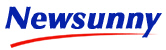首页 关于三菱 关于我们 联系三菱

GM-S F S B     （均一荷重用）
 GM：齿轮电机 S：S系列；SZ：变频定扭力 F：F=法兰安装；无=卧式 S：S=单相；无=三相 B：带刹车 ①：无=标准型；W=防水型（IP65）

GM-D F B     （中荷重用）
 GM：齿轮电机 D：D=D系列；DD=DD系列；DZ=V/F制御变频控制定扭力（防爆含 ）；DZ3=磁束制御变频控制定扭力；DT=V/F制御变频控制低减扭力（除防爆 ） F：F=法兰安装；无=卧式 B：带刹车 ①：无=标准型；A=安全增防爆型；C=防腐型；X：耐压防爆型；H=高性能省功型；W=防水型（IP65）
GM-LJ V B     （中荷重用）
 GM：齿轮电机 LJ：LJ=LJ系列；LLJ=LLJ系列；LJZ=变频器定扭力型 V：V=立式安装；无=卧式 B：B=带刹车（30～37KW）；B2=带刹车（3.7～22KW)；无=不带刹车 ①：无=标准型；A=安全增防爆型；C=防腐型；X：耐压防爆型

GM-J2 S E B     （均一荷重用）
 GM：齿轮电机 J2：J2系列 S：相数。无=三相；S=单相；R=单相可逆 E：E=速度可调；无=定速 B：带刹车 ①：T=带小端子箱；W=带大端子箱；无=无端子箱
GM-SY F B - R H    （均一荷重用）
 GM：齿轮电机 SY：SY=SY系列；SYZ=变频驱动定扭力系列 F：F=法兰安装，或面安装 B：带刹车 ①：无=标准型；W=防水型（IP65）；P=防水型（IP67） R：直交齿轮电动机 H：中空轴

GM-J2系列速度控制器
GC-U 90 1
 GC：速度控制器 U：U=尊贵型；无=标准型 90：90=90W以下；40=40W以下 1：1=100V用；2=200V用
GM-HY2 F B - R H    （中荷重用）
 GM：齿轮电机 HY2：HY2=HY2系列；HY2Z=变频驱动定扭力系列；HY=防水型 F：F=法兰安装；M=面安装；无=底座安装 B：带刹车 ①：无=标准型；W=防水型（IP65）；P=防水型（IP67） R：直交齿轮电动机 H：H=中空轴；L=左轴；R：右轴；T：两轴
GM-DY F B - R H    （中荷重用）
 GM：齿轮电机 DY：DY=DY系列；DYZ=变频驱动定扭力系列 F：F=法兰安装；M=面安装；无=底座安装 B：带刹车 R：直交齿轮电动机 H：H=中空轴；L=左轴；R：右轴
GV-S F S B - R H    （均一荷重用）
 GV：变频器内藏齿轮电动机 S：S=平行轴；HY2=直交轴 F：F=法兰安装；M=面安装；无=底座安装 S：相数。无=三相；S=单相 B：带刹车 R：直交齿轮电动机 H：H=中空轴；L=左轴；R：右轴；T：两轴